NCERT Solutions for Maths Class 12 Exercise 7.1

# NCERT Solutions for Maths Class 12 Exercise 7.1

## NCERT Solutions for Maths Class 12 Exercise 7.1

Hello Students! Welcome to maths-formula.com. In this post, you will find the complete NCERT Solutions for Maths Class 12 Exercise 7.1.

You can download the PDF of NCERT Books Maths Chapter 7 for your easy reference while studying NCERT Solutions for Maths Class 12 Exercise 7.1.

Class 12th is a very crucial stage of your student’s life, since you take all important decisions about your career on this stage. Mathematics plays a vital role to take decision for your career because if you are good in mathematics, you can choose engineering and technology field as your career.

NCERT Solutions for Maths Class 12 Exercise 7.1 helps you to solve each and every problem with step by step explanation which makes you strong in mathematics.

All the schools affiliated with CBSE, follow the NCERT books for all subjects. You can check your syllabus from NCERT Syllabus for Mathematics Class 12.

NCERT Solutions for Maths Class 12 Exercise 7.1 are prepared by the experienced teachers of CBSE board. If you are preparing for JEE Mains and NEET level exams, then it will definitely make your foundation strong.

If you want to recall All Maths Formulas for Class 12, you can find it by clicking this link.

If you want to recall All Maths Formulas for Class 11, you can find it by clicking this link.

NCERT Solutions for Maths Class 12 Exercise 7.2

NCERT Solutions for Maths Class 12 Exercise 7.3

NCERT Solutions for Maths Class 12 Exercise 7.4

NCERT Solutions for Maths Class 12 Exercise 7.5

NCERT Solutions for Maths Class 12 Exercise 7.6

NCERT Solutions for Maths Class 12 Exercise 7.7

NCERT Solutions for Maths Class 12 Exercise 7.8

NCERT Solutions for Maths Class 12 Exercise 7.9

NCERT Solutions for Maths Class 12 Exercise 7.10

NCERT Solutions for Maths Class 12 Exercise 7.11

## NCERT Solutions for Maths Class 12 Exercise 7.1

Find an antiderivative (or integral) of the following by the method of inspection in Exercises 1 to 5:

Maths Class 12 Ex 7.1 Question 1.

sin 2x

Solution:

Maths Class 12 Ex 7.1 Question 2.

cos 3x

Solution:

Maths Class 12 Ex 7.1 Question 3.

e2x

Solution:Maths Class 12 Ex 7.1 Question 4.

(ax + b

Solution:

Maths Class 12 Ex 7.1 Question 5.Solution:

Find the following integrals in Exercises 6 to 20:

Maths Class 12 Ex 7.1 Question 6.Solution:

Maths Class 12 Ex 7.1 Question 7.Solution:

Maths Class 12 Ex 7.1 Question 8.Solution:

Maths Class 12 Ex 7.1 Question 9.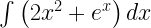Solution:

Maths Class 12 Ex 7.1 Question 10.Solution:

Maths Class 12 Ex 7.1 Question 11.Solution:

Maths Class 12 Ex 7.1 Question 12.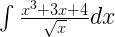Solution:

Maths Class 12 Ex 7.1 Question 13.Solution:

Maths Class 12 Ex 7.1 Question 14.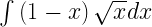Solution:

Maths Class 12 Ex 7.1 Question 15.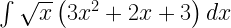Solution:

Maths Class 12 Ex 7.1 Question 16.Solution:

Maths Class 12 Ex 7.1 Question 17.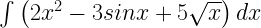Solution:

Maths Class 12 Ex 7.1 Question 18.Solution:

Maths Class 12 Ex 7.1 Question 19.Solution:

Maths Class 12 Ex 7.1 Question 20.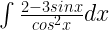Solution:

Choose the correct answer in Exercises 21 and 22.

Maths Class 12 Ex 7.1 Question 21.

Solution:

Maths Class 12 Ex 7.1 Question 22.